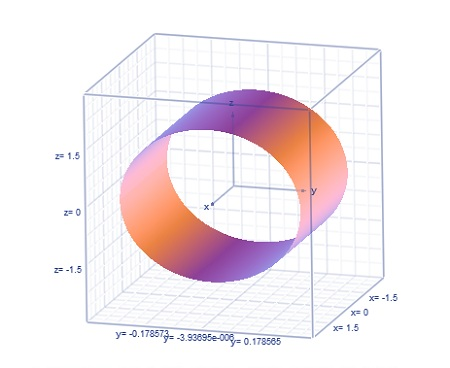# Describe the graph of the equation: 49y^2+z^2=4

## Question:

Describe the graph of the equation: {eq}49y^2+z^2=4{/eq}

## Graph:

The graph of the function in either the 3 dimensions or the 2 dimensions, will depend of the equation if it is in 2 variables or in three variables form.

The graphic calculator is used for such curve sketching.

## Answer and Explanation:

The graph of the equation is shown below:This the 3-dimensional cylinder.

#### Learn more about this topic:Graphs: Types, Examples & Functions

from High School Algebra II: Help and Review

Chapter 16 / Lesson 11
355K